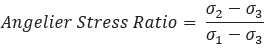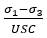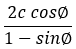# Principal Stress

The following principal stress data can be contoured.

### SIGMA 1:

Sigma 1 option will plot the contours of the MAJOR PRINCIPAL STRESS.

### SIGMA 3:

Sigma 3 option will plot the contours of the MINOR PRINCIPAL STRESS.

### SIGMA 2:

Sigma 2 is the intermediate principal stress which is always between Sigma 1 and Sigma 3.

### VON MISES STRESS:

The major, intermediate and minor principal stress corresponds to Sigma 1, Sigma 2 and Sigma 3 in EX3, respectively.

### ANGELIER STRESS RATIO:

The Angelier Stress Ratio is given by:where Sigma 1, Sigma 2 and Sigma 3 are the three-dimensional major intermediate and minor principal stress.

### SPALLING CRITERION:

The Spalling criterion is given by:where, USC is the uniaxial compressive strength. Noting that if the Mohr-Coulomb criterion is used to calculate the strength, this parameter can be calculated by:In which, c is the cohesion and ∅ is the friction angle.

### SIGMA3/SIGMA1:

Is a ratio of minor principle stress to the major principal stress